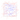19 February 2019 — written by Prakash Prasad

This is a shader test to see if the glslify pluginf or gatsby can render shader files and how they look...

# Prime Number Theorem

22 March 2009 — written by Prakash Prasad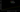Prime numbers are a fascinating topic that has been studied since ancient Greeks due to interesting characteristics. Prime number theorem is one such topic that aims to estimate the density of prime numbers for any given number...

# N Queens Problem

19 March 2009 — written by Prakash Prasad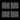The standard N Queen’s problem asks a very interesting question – how can N queens be placed on an N x N chess board in such a way so that none of them can hit any other in their turn?...

# Mandelbrot Set

17 March 2009 — written by Prakash Prasad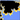Mandelbrot set was named after the mathematician Benoit Mandelbrot, and its a set of numbers drawn on the complex number plane to create a complex 2D geometry from a very simple mathematical equation...

# Magic Squares

15 March 2009 — written by Prakash Prasad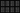A 'Magic Square' is an arrangement of the distinct positive integers 1, 2, 3, …, n^2 such that the sum of all the rows, columns and main diagonals is always the same number, which is known as the 'Magic Constant'. Lets try to write an algorithm that can find a magic square for N x N and see what kind of performance we can get to ...